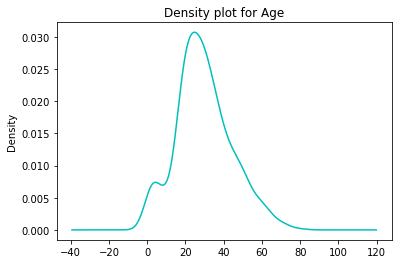top of page
Search

# Titanic Exploratory Data Analysis### Would You Survive The Titanic?

The Titanic Ship incident is very much well known to everyone. Here in this blog, we are going to discuss the titanic data with a detailed analysis.

The dataset is present in the Kaggle itself.

### Dataset Description:

This dataset contains 891 rows with 12 columns.

The column descriptions are shown below### Column description:

pclass: A proxy for socio-economic status (SES)

1st = Upper

2nd = Middle

3rd = Lower

age: Age is fractional if less than 1. If the age is estimated, is it in the form of xx.5

sibsp: The dataset defines family relations in this way...

Sibling = brother, sister, stepbrother, stepsister

Spouse = husband, wife (mistresses and fiancés were ignored)

parch: The dataset defines family relations in this way...

Parent = mother, father

Child = daughter, son, stepdaughter, stepson

Some children traveled only with a nanny, therefore parch=0 for them.

survival: It defines a person present in the ship was Survived or not.

Age: Indicates a person's age in years.

ticket: Ticket number of the person.

fare: Passenger fare

cabin: Cabin number

embarked: Port of Embarkation (C = Cherbourg, Q = Queenstown, S = Southampton)

The dataset's first 5 rows are shown below## Data Analysis:This figure has shown the survived column where 0 represents NO and 1 represents YES.This figure has shown the sex column where we can see that males numbers were greater than female ones.This figure has shown the survived column wrt the sex column, where we can see that males deaths are more than female ones whereas female survival rate is more than male ones.This figure showed the age distribution of all the ages. It is shown that between the age group (20-35) most of the passengers are present.

we can also plot by changing the size of the bins.

```# use bins to add or remove bins
titanic_data.Age.plot(kind='hist', title='histogram for Age', color='c', bins=20);```For KDE(Kernel Density Estimation plot) we have to plot using KDE.

```# use kde for density plot
titanic_data.Age.plot(kind='kde', title='Density plot for Age', color='c');```Now let's plot the scatter points between age and fare column.Plot with more TransparencyThe below figure shows the distribution of passenger's class. so from this figure we can see that the age group between(30-50) traveled in Pclass:1, the age group between(25-30) traveled in Pclass:2, and mostly the Youths were traveled in Pclass:3.### Data Wrangling and Preprocessing:

In this section, we will discuss how to handle with NAN values.

`sns.heatmap(titanic_data.isnull(),cmap='Accent')`This heatmap shows the NAN Values present in the dataset. we can simply drop The column contains most of the NAN values i.e. Cabin.

`titanic_data.drop(['Cabin'],axis=1,inplace=True)`

So now from the titanic data cabin column has been removed.

Now the age column has also contained the NAN values, so we can do drop all the NAN values, or we can fill it by some numbers.

Things to be done

1. Dropping values can pamper our dataset cleaning.

2. Filling random values is still not a good choice.

3. Filling by the mean age column is a good choice but we have to check the filling values to each data.

`titanic_data.groupby(['Pclass'])['Age'].mean()`

Output:

P-class 1 38.233441 2 29.877630 3 25.140620 Name: Age, dtype: float64

Here we have to fill age.mean() for the 3 Pclasses.

Now we have to return each age.mean() to the respective Pclass.

Now let's check for the Embarked column. it contains two NAN values. so we can fill the NAN values to the most repeated occurrence.

Let's check the most repeated occurrence.

`titanic_data['Embarked'].value_counts()`

Output:

S 644 C 168 Q 77 Name: Embarked, dtype: int64

So S is the most repeated occurrence so we can replace the two NAN values by S.

After replacing let's check if our dataset contains any further NAN values.

`sns.heatmap(titanic_data.isnull())`So we have ready with the dataset to fit in our model.

The last step is to be converting categorical variables into numerical ones, and drop unnecessary columns. Here we have two categorical columns i.e. Sex and embarked.

so first drop the unnecessary columns.

`titanic_data['Embarked'].replace(to_replace=['S','C','Q'],value=['1','2','3'],inplace=True)`

Now the embarked column has been changed to numerical ones. we are now left with Sex column.

```from sklearn import preprocessing
# label_encoder object knows how to understand word labels.
label_encoder = preprocessing.LabelEncoder()
titanic_data['Sex']= label_encoder.fit_transform(titanic_data['Sex']) ```

Now the sex column also has been changed to numerical ones.

The Analysis has been made to each column present in the titanic dataset. Now it can be directly fit into the model for prediction.

Thank You!